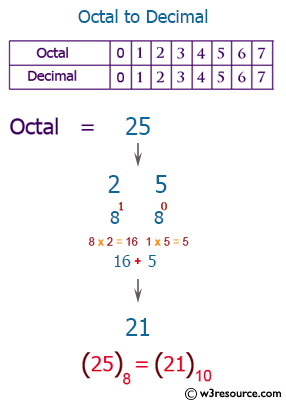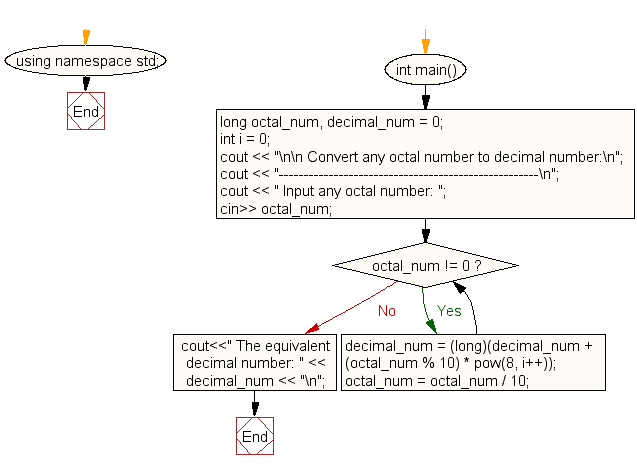﻿ C++ Exercises: Convert a octal number to decimal number - w3resource

# C++ Exercises: Convert a octal number to decimal number

## C++ For Loop: Exercise-76 with Solution

Write a C++ program to convert an octal number to a decimal number.

Pictorial Presentation:Sample Solution:-

C++ Code :

``````#include <iostream>
#include <math.h>
using namespace std;

int main()
{
long octal_num, decimal_num = 0;
int i = 0;
cout << "\n\n Convert any octal number to decimal number:\n";
cout << "----------------------------------------------------\n";
cout << " Input any octal number: ";
cin>> octal_num;
while (octal_num != 0)
{
decimal_num = (long)(decimal_num + (octal_num % 10) * pow(8,

i++));
octal_num = octal_num / 10;
}
cout<<" The equivalent decimal number: " << decimal_num << "\n";
}
``````

Sample Output:

``` Convert any octal number to decimal number:
----------------------------------------------------
Input any octal number: 17
The equivalent decimal number: 15
```

Flowchart:C++ Code Editor: